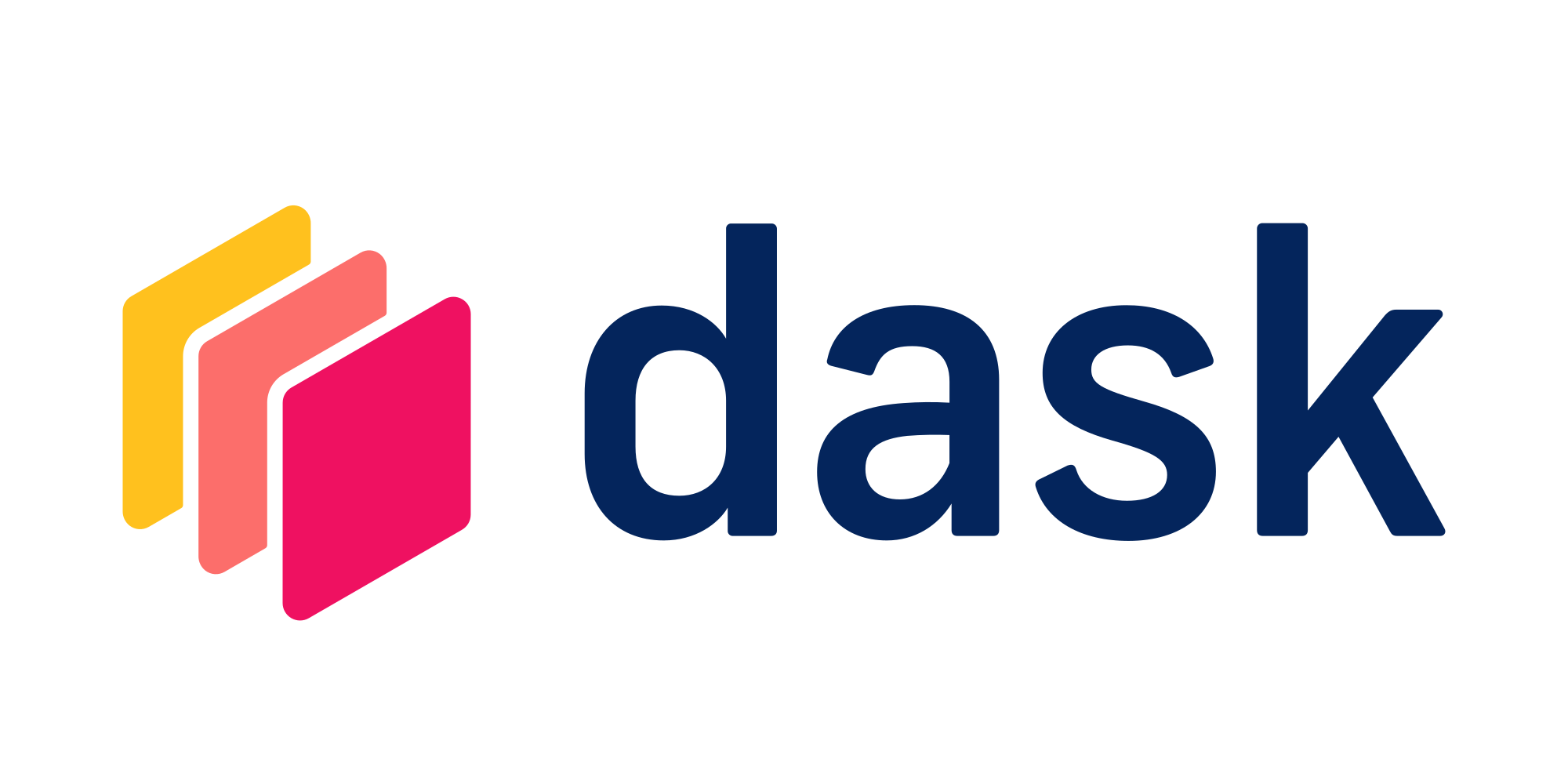# Rolling Averages

Rolling average calculations with data that is distributed over a Dask clusterTry this example in seconds on Saturn Cloud

I need to calculate a rolling average of a numerical column, in time series data. In pandas, I can do this with rolling(x).mean() with sorted values, but what do I do in Dask, with distributed data?

This example will walk you through performing rolling average calculations with data that is distributed over a Dask cluster. We’ll use New York City taxi trip data and get the 30-day rolling average of base fare prices for our example.

``````from dask_saturn import SaturnCluster

client = Client(SaturnCluster())
``````

After running the above command, it’s recommended that you check on the Saturn Cloud resource page that the Dask cluster as fully online before continuing. Alternatively, you can use the command `client.wait_for_workers(3)` to halt the notebook execution until all three of the workers are ready.

Now load your data into a Dask DataFrame. Here we are loading data for parquet file located in a public location hosted by Saturn Cloud. Using `read_parquet` from Dask takes the same form as using that function from pandas.

``````import dask.dataframe as dd

"s3://saturn-public-data/nyc-taxi/data/yellow_tripdata_2019-01.parquet",
storage_options={"anon": True},
).sample(frac=0.1, replace=False)
``````

For the DataFrame we are calling function `set_index` to set `tpep_pickup_datetime` columns as the index. This will sort data by index within and across partitions. At this point, data and labels are lazy collections. They won’t be read into the workers' memory until some other computation asks for them. Hence, before we pass this data to other tasks we will call `persist()`. This will ensure that loading of data is run only once.

``````taxi = taxi.set_index("tpep_pickup_datetime")
taxi = taxi.persist()
``````

In code below we get the 30-day rolling average of base fare prices ie. on column `fare_amount` and convert the resulting series to DataFrame.

``````rolling_fares = taxi.fare_amount.rolling("30D").mean()
rolling_fares_df = rolling_fares.to_frame(name="fare_amount_rolled")
``````

We then join the original DataFrame `taxi` with DataFrame `rolling_fares_df`.

``````taxi_new = taxi.join(rolling_fares_df, how="outer")
``````

The rolling average computation is now complete. This is how the first few lines of rolling average look like in `taxi_new` DataFrame!

``````from dask_saturn import SaturnCluster

client = Client(SaturnCluster())

"s3://saturn-public-data/nyc-taxi/data/yellow_tripdata_2019-01.parquet",
storage_options={"anon": True},
).sample(frac=0.1, replace=False)

taxi = taxi.set_index("tpep_pickup_datetime")
taxi = taxi.persist()

rolling_fares = taxi.fare_amount.rolling("30D").mean()
rolling_fares_df = rolling_fares.to_frame(name="fare_amount_rolled")

taxi_new = taxi.join(rolling_fares_df, how="outer")

``````